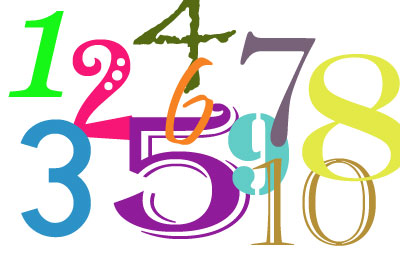#### You may also like### Pebbles

Place four pebbles on the sand in the form of a square. Keep adding as few pebbles as necessary to double the area. How many extra pebbles are added each time?### Bracelets

Investigate the different shaped bracelets you could make from 18 different spherical beads. How do they compare if you use 24 beads?### Sweets in a Box

How many different shaped boxes can you design for 36 sweets in one layer? Can you arrange the sweets so that no sweets of the same colour are next to each other in any direction?

# Abundant Numbers

##### Age 7 to 11Challenge LevelTo find the factors of a number, you have to find all the pairs of numbers that multiply together to give that number.

The factors of $48$ are:

$1$ and $48$

$2$ and $24$

$3$ and $16$

$4$ and $12$

$6$ and $8$

If we leave out the number we started with, $48$, and add all the other factors, we get $76$:

$1 + 2 + 3 + 4 + 6 + 8 + 12 + 16 + 24 = 76$

So .... $48$ is called an abundant number because it is less than the sum of its factors (without itself). ($48$ is less than $76$.)

See if you can find some more abundant numbers!